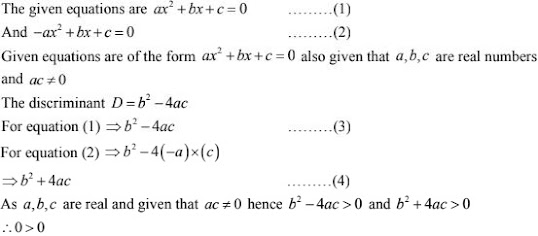# RD Sharma Solutions Chapter 8 Quadratic Equations Exercise 8.6 Class 10 Maths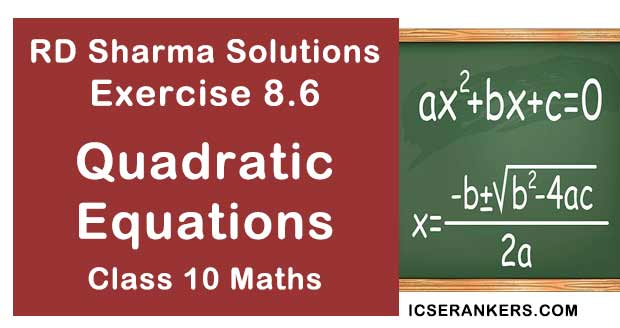Chapter Name RD Sharma Chapter 8 Quadratic Equations Book Name RD Sharma Mathematics for Class 10 Other Exercises Exercise 8.1Exercise 8.2Exercise 8.3Exercise 8.4Exercise 8.5Exercise 8.7Exercise 8.8Exercise 8.9Exercise 8.10Exercise 8.11Exercise 8.12Exercise 8.13 Related Study NCERT Solutions for Class 10 Maths

### Exercise 8.6 Solutions

1. Determine the nature of the roots of the following quadratic equations:

(i) 2x2 – 3x + 5 = 0
(ii) 2x2 - 3x + 5 = 0
(iii) (3/5)x2 - (2/5)x + 1 = 0
(iv) 3x2 - 4√3x + 4 = 0
(v) 3x2 - 2√6x + 2 = 0
(vi) (x - 2a)(x - 2b) = 4ab
(vii) 2(a2 + b2 )x2 + 2(a + b)x + 1 = 0
(viii) 2(a2 + b2 )x2 + 2(a + b)x + 1 = 0
(ix) (b + c)x2 - (a +b +c)x + a = 0

Solution

(i) 2x2 – 3x + 5 = 0
The given quadratic equation is 2x2 – 3x + 5 = 0
here a = 2, b = -2, c = 5
D = b2 + 4ac
⇒ (-3)2 - 4 ×5 ×1 = 9 -20 = -11 < 0
As D < 0, The discriminant of equation is negative, then the expression has no real roots

(ii) 2x2 - 3x + 5 = 0
The given quadratic equation is 2x2 - 3x + 5 = 0
here a = 2, b = -6 and c = 3
∴ D = b2 - 4ac
⇒ (-6)2 - 4×2×3 = 36 - 24 = 12 > 0
As D > 0, the discriminant of equation is positive, the equation has real and distinct roots

(iii) (3/5)x2 - (2/5)x + 1 = 0
The given quadratic equation is (3/5)x2 - (2/3)x + 1 = 0  can also be written as 9x2 - 10x + 15 = 0
here a = 9, b = -10, c = 15
D = b2 - 4ac
⇒ (-10)2 - 4×5×9 ⇒ 100 - 540 = -440 < 0
∴ as D > 0, the equation has no real roots

(iv) 3x2 - 4√3x + 4 = 0
The given quadratic equation is 3x2 - 4√3x + 4 = 0
here a = 3, b = -4√3, c = 4
The discriminant D = b2 - 4ac
⇒ (-4√3)2 - 4×3×4 = 48 - 48 = 0
as D > 0, the equation has real and equal roots

(v) 3x2 - 2√6x + 2 = 0
The given quadratic equation is 3x2 - 2√6x + 2 = 0
Here, The equation is in the form of ax2 + b + c = 0
Where a = 3, b = -2√6 and c = 2
D = b2 - 4ac
⇒ (-2√6)2 - 4×3×2 = 24 - 24 = 0
as D = 0, the given quadratic equation has real and equal roots

(vi) (x - 2a)(x - 2b) = 4ab
The given equation (x - 2a)(x - 2b) = 4ab  can also be written as x2 - x(2a + 2b) and
c = 0[4ab - 4ab = 0 ]
D = b2 - 4ac
⇒ [-(2a + 2a)]2 - 4×1×0 = (2a + 2b)2 > 0
⇒ as equal root of any integers is always positive
⇒ D > 0, hence the discriminant of the equation is positive

(vii) 2(a2 + b2 )x2 + 2(a + b)x + 1 = 0
The given equation is 2(a2 + b2 )x2  + 2(a + b)x + 1 = 0
It is in the form of the equation ax2 + bx + c = 0
Here a = 2(a2 + b2 ),  b = 2(a + b) and c = 1
∴ D = b2 - 4ac
⇒ (-2abcd)2 - 9×9a2b2 × 16c2d2
⇒ b + 6a2 b2 c2 d2 - 57a2 b2 c2 d2 = 0
Hence as D = 0, the equation as Real and equal roots

(viii) 2(a2 + b2 )x2 + 2(a + b)x + 1 = 0
The given equation is 2(a2 + b2 )x2 + 2(a + b)x + 1 = 0
It is in the farm of the equation ax2 + bx + c = 0
Here a = 2(a2 + b2 ), b = 2(a + b) and c = 1
∴ D = b2 - 4ac
⇒ [2(a + b)]2 - 4 × 2(a2 + b2 )×1
⇒ 4a2 + ab2 + 8ab - 8a2 - 8b2
⇒8ab + 4(a2 + b2 ) < 0
as D < 0, The discriminant is negative and the nature of the roots are not real

(ix) (b + c)x2 - (a + b + c)x + a = 0
The given equation is (b + c)x2 - (a + b +c)x + a = 0
Here a + b + c, b = -(a + b + c) and c = a
∴ D = b2 - 4ac
⇒ [ - (a + b + c)]2 - 4×(b+c)×a
⇒(a + b +c)2 - 4abc > 0
∴ as D > 0, the discriminant is positive and the nature of the roots are real and unequal

2. Find the values of k for which the roots are real and equal in each of the following equation:

(i) kx2 + 4x + 1 = 0
(ii) kx2 - 2√5x + 4 = 0
(iii) 3x2 - 5x + 2k = 0
(iv) 4x2 + kx + 9 = 0
(v) 2kx2 - 40x + 25 = 0
(vi) 9x2 - 24x + k = 0
(vii)4x2 - 3kx + 1 = 0
(viii) x2 - 2(5 + 2k)x + 3(7 + 10k) = 0

Solution

(i) kx2 + 4x + 1 = 0
The given equation  kx2 + 4x + 1 = 0 is in the form of  ax2 + bx + c = 0 where
a =  k, b = 4, c = 1
given that, the equation has real and equal roots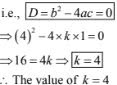(ii) kx2 - 2√5x + 4 = 0

The given equation kx2 - 2√5x + 4 = 0 is in the form of ax2 + bx + c = 0 where
a = k, b = -2√5 and c = 4
⇒ given that, the equation has real and equal roots
i.e., D = b2 - 4ac = 0
⇒ (-2√5)2  4 ×k ×4 = 0
⇒  20 = 16k ⇒ k = 20/16 = 5/4 ∴ k = 5/4
∴ The value of k = 5/4

(iii) 3x2 - 5x + 2k = 0

The given equation is 3x2 - 5x + 2k = 0
This equation is in the form of ax2 + bx + c = 0
Here, a = 3, b = -5 and c = 2k
⇒given that, the equation has real and equal roots
i.e., D = b2 - 4ac = 0
⇒ (-5)2 - 4×3×(2k) = 0
⇒ 25 = 24k
⇒ k = 25/24
∴ The value of k = 25/24

(iv) 4x2 + kx + 9 = 0

The given equation is 4x2 + kx + 9 = 0
This equation is in the form of ax2 + bx + c = 0
Here, a = 4, b = k and c = 9
given that, the equation has real and equal roots
i.e., D = b2 - 4ac = 0
⇒ k2 - 4×4×9 = 0
⇒ k2 - 16×9
⇒ k = √(16×9) = 4×3 = 12
∴ The value of k = 12

(v) 2kx2 - 40x + 25 = 0

The given equation is 2kx2 - 40x + 25 = 0
This equation is in the form of ax2 + bx + c = 0
Here, a = 2k, b = -40 and c = 25
Given that, the equation has real and equal roots
I.e., D = b2 - 4ac = 0
⇒ (-10)2 - 4×2k ×25 = 0
⇒ 1600 - 200k  = 0
⇒ k = 1600/200 = 8    ∴ k = 8
∴ The value of k = 8

(vi) 9x2 - 24x + k = 0

The given equation is 9x2 - 24x + k = 0
This equation is in the form of ax2 + bx + c = 0
Here, a = 9, b = -24, and c = k
given that, the nature of the roots of this equation is real and equal
i.e., D = b2 - 4ac = 0
⇒(-24)2 - 4×4×k = 0
⇒ 576 - 36k = 0
⇒ k = 576/36 = 16     ∴ k = 16
∴ The value of k = 16

(vii) 4x2 - 3kx + 1 = 0

The given equation is 4x2 - 3kx  + 1 = 0
This equation is in the form of ax2 + bx +c = 0
Here, a = 4, b = -3k, and c = 1
given that, the nature of the roots of this equation is real and equal
i.e., D = b2 - 4ac = 0
⇒ D = b2 - 4ac = 0
⇒ (-3k)2 - 4×4×1 = 0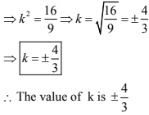(viii) x2 - 2(5 + 2k)x + 3(7 + 10k) = 0

The given equation is x2 - 2(5 + 2k)x + 3(7 + 10k) = 0
Here, a = 1, b = -2(5+2k) and c = 3(7 + 10k)
given that, the nature of the roots of this equation are real and equal
i.e., D = b2 - 4ac = 0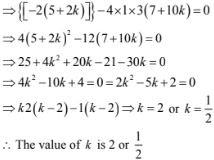(ix) (3k + 1)x2 + 2(k + 1)x + k = 0

The given equation is (3k + 1)x2 + 2(k + 1)x + k = 0
This equation is in the form of ax2 + bx + c = 0
Here a =3k + 1, b = 2(k + 1) and  c = k
Given that the nature of the roots of this equation are real and equal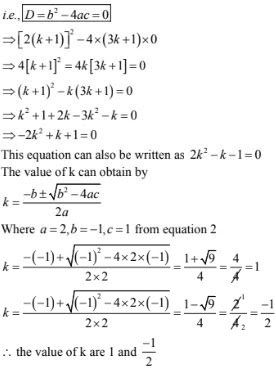(x) kx2 + kx + 1 = -4x2 - x

The given equation is kx2 + kx + 1 = -4x2 - x bringing all the 'x' components to one side,
we get the equation as x2 (4 +k) + x(k + 1) + 1 = 0
This equation is in the form of the general quadratic equation i.e., ax2 + bx + c = 0
Here a = 4 + k, b = k + 1 and c = 1
given that the nature of the roots of the given equation are real and equal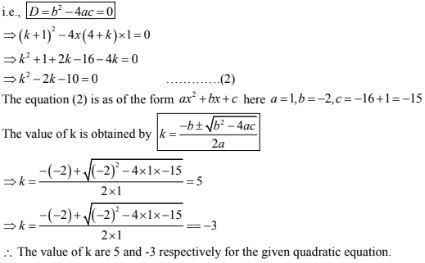(xi) (k + 1)x2 + 20 = (k + 3)x + (k + 8) = 0
The given equation is (k + 1)x2 + 2(k + 3)x + (k + 8) = 0
Here a = k + 1, b = 2(k + 3) and c = k + 8
given that the nature of the roots of this equation are real and equal i.e.,
D = b2 - 4ac = 0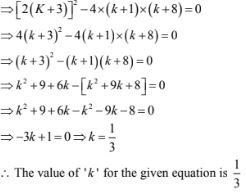(xii) x2 -2kx +7k -12 = 0
The given equation is x2 -2kx +7k -12 =0
Here a = 1, b = -2k and c = 7k -12
given that the nature of the roots of this equation are real and equal i.e.,
D = b2 - 4ac = 0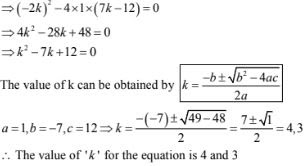(xiii) (k +1)x2 - 2(3k + 1)x + 8k +1 = 0
The given equation is (k +1)x2 - 2(3k +1)x + 8k +1 =0
It is in the form of the equation ax2 + bx + c = 0
Here, a = k +1, b = -2(3k + 1) and c = 8k +1)
given that the nature of the roots of the given equation are real and equal i.e.,
D = b2 - 4ac = 0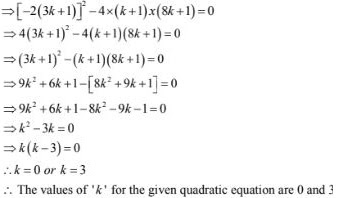(xiv) 5x2 - 4x +2 +k(4x2 - 2x +1) = 0
The given equation is 5x2 -4x +2 +k(4x2 - 2x +1) = 0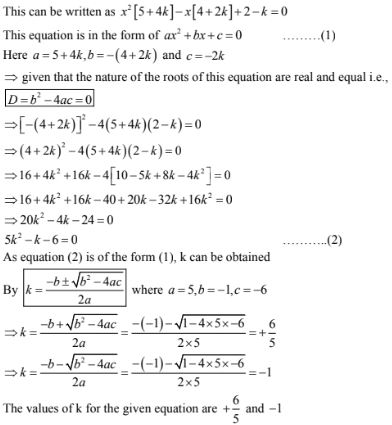(xv) (4 - k)x2 + (2k + 4)x + (8k + 1 ) = 0
The given equation is (4 - k)x2 + (2k + 4)x + (8k + 1)= 0
This equation is in the form of ax2 + bx + c = 0
Here a = 4 - k, b = 2k + 4 and c = 8k + 1
given that the nature of the roots of this equation are real and equal i.e.,
D = b2 - 4ac = 0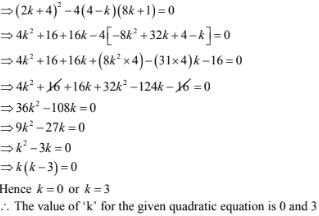(xvi) (2k + 1)x2 + 2(k + 3)x + (k + 5) = 0
The given equation is (2k + 1)x2 + 2(k + 3)x + (k + 5) = 0
This equation is in the form of ax2 + bx + c = 0
Here a = 2k + 1, b = 2(k + 3) and c = k + 5
given that the nature of the roots for this equation are real and equal i.e.,
D = b2 - 4ac = 0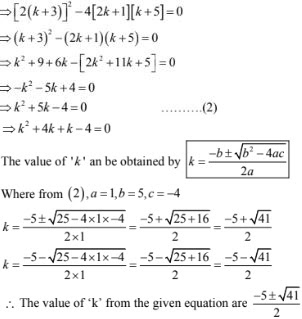(xvii) 4x2 - 2(k + 1)x + (k + 4) = 0
The given equation is 4x2 - 2(k + 1)x  + (k + 4) = 0
This equation is in the form of ax2 + bx + c = 0
here a = 4, b = -2(k + 1), c = k + 4
Given that the nature of the roots of this equation is real equal i.e. D = b2 - 4ac = 0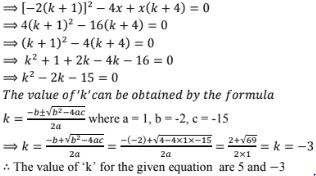(xviii) x2 - 2(k + 1)x + (k + 4) = 0
The given equation is x2 - 2(k + 1)x  + (k + 4) = 0
This equation is in the form of ax2 + bx + c = 0
Here a = 1, b = -2(k + 1) and c = k + 4
The nature of the roots of this equation is given that it is real and equal
i.e., O = b2 - 4ac = 0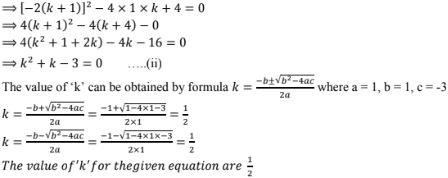(xix) 4x2 - 2(k + 1)x + 4 = 0
The given equation as k2x2 - 2(2k - 1)x + 4 = 0
It is in the form of the equation ax2 + bx + c = 0
Here a = k2 , b = -2(2k - 1) and c = 4
Given that the nature of the roots of the equation are real and equal
i.e., D = b2 - 4ac = 0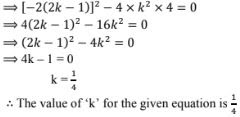(xx) (k + 1)x2 - 2(k - 1)x + 1 = 0
The given equation is (k + 1)x2 - 2(k - 1)x + 1 = 0
It is in the form of the equation ax2 + bx + c = 0
Here a = k + 1, b = -2(k - 1) and c = 1
given that the nature of the roots for the equation are real and equal
i.e., D = b2 - 4ac = 0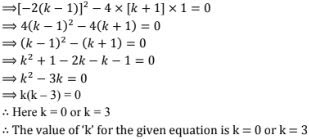(xxi) 2x2 + kx + 3 = 0
The given equation is 2x2 + kx + 3 = 0
It is in the form of the equation ax2 + bx +c = 0
Here a = 2, b = k, and c = 3
given that the roots of the equation are real and equal i.e., D = b2 - 4ac = 0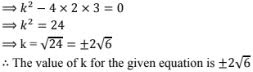(xxii) kx(x - 2) + 6 = 0
The given equation is kx2 -2kx + 6 = 0
a = 6, b = - 2k, c = 6
given that the roots are real and equal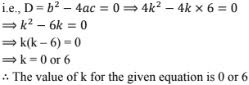(xxiii) x2 - 4kx + k = 0
The given equation is x2 - 4kx + k = 0
a = 1, b = -4k, c = k
given that the roots are real and equal i.e.,
D = b2 - 4ac = 0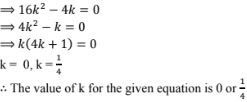3. In the following determine the set of values of k foe which the green quadratic equation has real roots:
(i) 2x2 + 3x + k = 0
(ii) 2x2 + kx + 3 = 0
(iii) 2x2 - 5x - k = 0
(iv) kx2 + 6x +1 = 0
(v) x2 -kx + 9 = 0
Solution
(i) 2x2 + 3x + k = 0
The given equation is 2x2 + 3x + k = 0
given that the quadractic equation has real roots i.e., D = b2 - 4ac ≥ 0
Given here a = 2, b = 3, c = k
⇒ 9 - 4 ×2×k ≥ 0
⇒ 9 - 8k ≥ 0
⇒ 9 ≥ 8k
⇒ k ≤ 9/8
The value of k does not exceed 4/8 to have roots

(ii) 2x2 + kx + 3 = 0
The given equation is 2x2 + kx + 3 = 0
given that the quadratic equation has real roots i.e., D = b2 - 4ac ≥ 0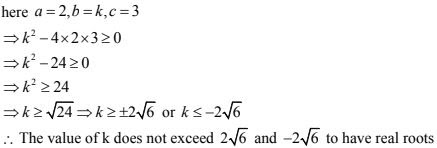(iii) 2x2 - 5x - k = 0
The given equation is 2x2 - 5x - k = 0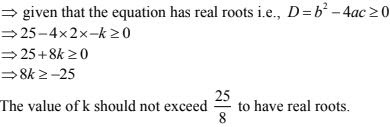(iv) kx2 + 6x + 1 = 0
The given equation is kx2 + 6x + 1 = 0
Here a = k, b = 6, c = 1
given that the equation has real roots
i.e., D = b2 - 4ac ≥ 0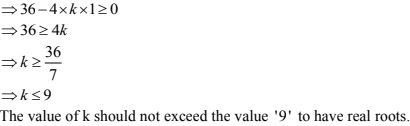(v) x2 - kx + 9 = 0
The given equation is x2 - kx + 9 = 0
Here a = 1, b = - k, c = 9
given that the equation has real roots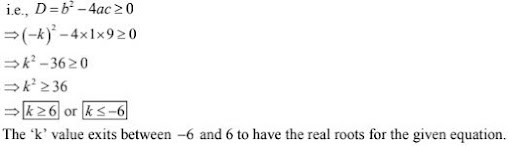(vi) 2x2 + kx + 2 = 0
The given equation is 2x2 + kx + 2 = 0
Here a = 2, b = k, c = 2
Given, that the equation has real roots
i.e., D = b2 - 4ac ≥ 0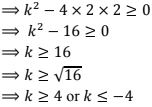∴ The k value lies between -4 and 4 to have the real roots for the given equation.

(vii) 3x2 + 2x + k = 0
The given equation has 3x2 + 2x + k = 0
Here a = 3, b = 2, c = k
Given that the quadratic equation has real roots i.e., D= b2 - 4ac
⇒ 4 - 4 ×3 × k ≥ 0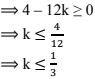The 'k' value should not exceed 1/3 to have the real roots for the given equation.

(viii) 4x2 - 3k + 1 = 0
The given equation has 4x2 - 3k + 1 = 0
Here a = 4, b = -3k, c = 1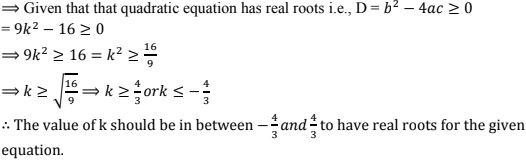(ix) 2x2 + kx - 4 = 0
The given equation is 2x2 + kx  - 4 = 0
Here a = 2, b = k, c = - 4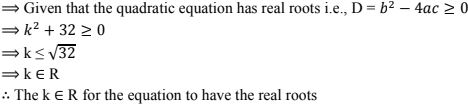4. For what value of k, (4 - k)x2 + (2k + 4)x + (8k + 1) = 0,  is a perfect square.
Solution
The given equation is (4 - k)x2 + (2k + 4)x + (8k + 1) = 0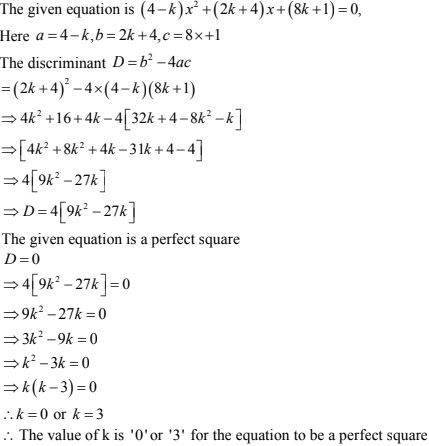5. Find the least positive value of k for which the equation x2 + kx + 4 = 0 has real roots.
Solution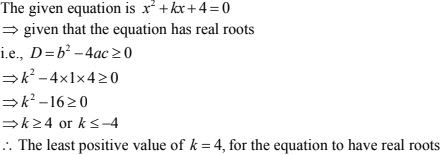6. Find the value of k for which the gives quadratic equation has real and distinct roots
(i) kx2 + 2x + 1 = 0
(ii) kx2 + 6x + 1 = 0
(iii) x2  - kx + 9 = 0
Solution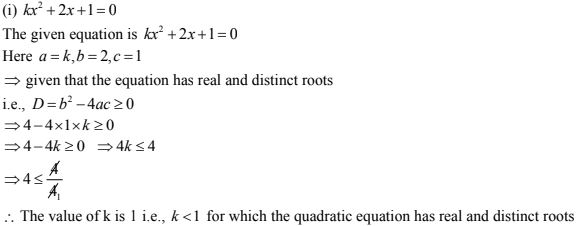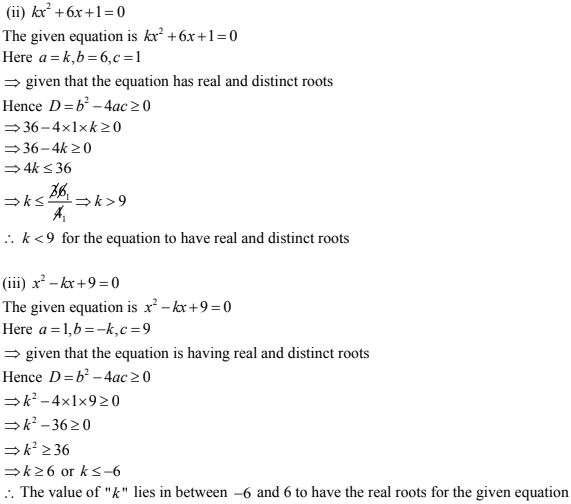7. If the roots of the equation (b - c)x2 + (c - a)x + (a -b) = 0 are equal, then prove that
2b = a + c
Solution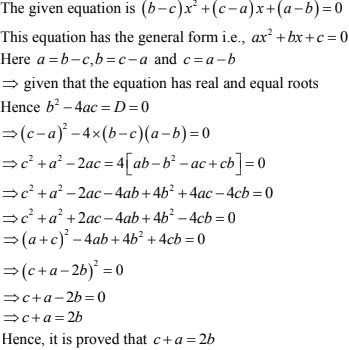8. If the roots of the equation (a2 + b2 )x2 - 2(x + bd)x + (c2 + d2 ) = 0 are equal, prove that
a/b = c/d
Solution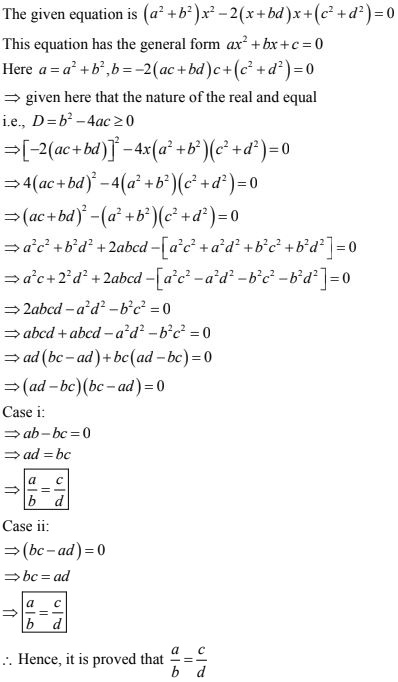9. If  the roots the equation ax2 + 2bx + c = 0 and bx2 - 2√cax + b = 0  are simultaneously real, then prove that b2 - ac.
Solution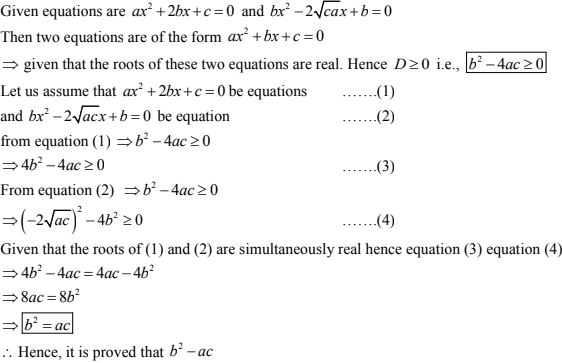10. If p, q are real and p ≠ q, then show that the roots of the equation  (p - q)x2  + 5(p + q)x - 2(p - q) - 0 are real and unequal
Solution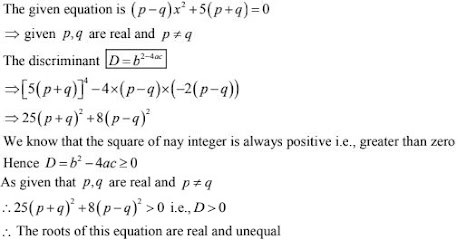11. If the roots of the equation(c2 - ab)x2 - 2(a2 - bc)x + b2 - ac = 0 are equal, prove that
either a = 0 or a3 + c3 = 3abc.
Solution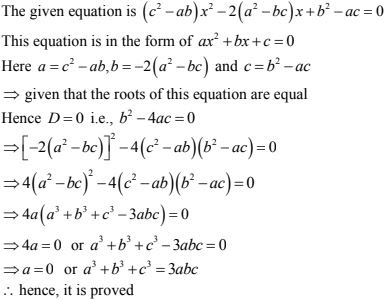12. Show that the equation 2(a2 + b2 )x2 + 2(a + b)x + 1 = 0 has no real roots, when a ≠ b.
Solution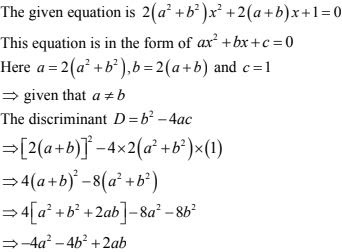As given that a ≠ b, as the discriminant 0 has negative squares, 0 will be less that zero
Hence 0 < 0, when a ≠ b

13. Prove that both the roots of the equation  (x - a)(x - b)+ (x - b)(x - c)+ (x - c)(x - a) = 0
are real but they are equal only when a = b = c.
Solution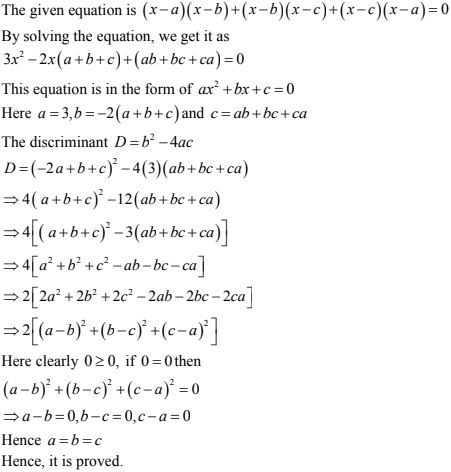14. If a, b, c are real numbers such that ac ≠ 0, then show that at least one of the equations ax2 + bx + c = 0  and  -ax2 + bx + c = 0 has real roots
Solution